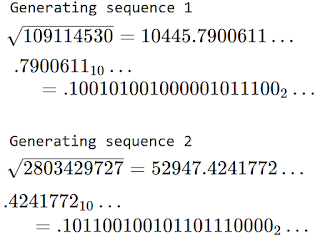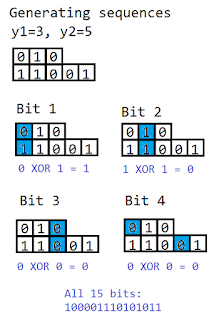## Wednesday, 14 February 2018

### Freakazoid, a Repetition-Robust Symmetric Cipher.

This post describes the mathematical principles behind the Freakazoid cipher such that someone could recreate it with software if they wished. For a targeted guide to some of the terminology used here, please see my previous post discussing some fundamentals of encryption.

It is not meant to be a serious attempt at a cipher, and is presented as food for thought only. It was originally developed more than ten years ago as part of a semester of research as a math undergrad.

Freakazoid is a cipher based on DES, an old cipher that was used as an industry standard for a time, but with the added step that a new key is generated each block of data to be encrypted. The generation of this key is governed by a master key. For clarity, we call a key applied to a particular block of data the block key.

Generation of Block Keys

Step A1 – Select a set of 'generating numbers' using the master key. In the implementation that was tested, a master key of 192 bits generated 12 such numbers by computing

2^x_1 * 3^x_2 * 5^x_3 * … * 47^x_15 53^x_16 , where  x_k  is the kth bit out of 16 bits from the master key dedicated to this particular generating number, and where the sequence (2,3,5,7,11, … , 47,53) is the first 16 primes.

See Figure 1 for an illustration of this for two generating numbers.Figure 1 - Step A1

Step A2 – For i=1,2,...,12. Compute the square root of the ith generating number to y_i bits of precision beyond the whole number. In this case, y_i is the ith prime number greater than 100.

Note that in most systems, a floating point sqrt() operating cannot provide this much precision, so an array based method adapted from the iterated subtraction method shown in this NIST document was used instead.

The non-integral bits from each of the generating numbers are used to produce the block keys.Figure 2 - Step A2
Step A3 – To generate a block key of 64 bits, take a consecutive sequence of 64 bits from each of the 12 square root sequences, wrapping around to the beginning of each sequence if the end is reached.

After 12 sequences of 64 bits each are collected, update the starting points in the sequences for the next block. For each of the 12 starting points s in the sequence, for each new block, apply (s + 64) mod y_i to update the ith starting point.

For the first block, take the first 64 bits from each of the square root sequences. This is the only time in billions of blocks that the starting points will be aligned so. Every starting point is updated to bit #65.

For the second block, the bits takes longer to describe.

We would attempt to take bits 65-128 from the first sequence, but since y_1 = 101, that sequence is only 101 bits long. So we take bits 65-101 and 1-27 instead. The first starting point is updated to bit 28.

Likewise, we would take bits 65-103 and 1-25 from the second sequence. Starter s_2 would be updated to be 26.

We would take bits 65-107 and 1-21 from the third sequence. S_3 would be updated to 22.

After two blocks, the starting points would be 28, 26, 22, 16, 10, 2, 65, 65, 65, 65, 65 and 65.
After four or more blocks, the starting points would be completely chaotic.

See Figure 3 for an illustration of Steps A3 and A4 for a simpler case of two sequences of length 3 and 5, respectively.

Step A4 – Addition Step

The nth bit of the block key is the XOR sum of the nth bits of each of the 64-bit sequences.Figure 3 - Steps A3 and A4

Use of Block Keys for Encryption

These block keys are used in two encryption methods similar to those in DES.

Step B1 – Addition Step

The block key is added to the plaintext bit-by-bit with the XOR operation.

Step B2 – Rearrangement Step

There are 8! = 5040 ways to permute the 8 bytes that make up a block. One of these 5040 arrangements is selected by taking the first 16 bits of the block key as P, and finding P mod 5040.

The bytes of the block are rearranged according to that permutation.

Step B3 – Substitution Step

The 64-bit block is now broken up into 4-bit nibbles. Each of these nibbles can only take on 1 of 16 possible values (0000, 0001, 0010, … ,1110, 1111).

A substitution table is created to replace every nibble of one value with a nibble of another value. For example, if the permutation of 1-16 had '8' in the 3rd entry, then every 3 or 0011 would be replaced by an 8 or 1000.

Every nibble is substituted according to the table exactly once.

There are 16! ~ 2.1 * 10^13 ways to set up such a table with a permutation of the integers 0 to 15, and we determine which one is used by taking the modulo 16! of the remaining 48 bits that were not used in selecting the rearrangement permutation.

The block output from step B3 is our ciphertext.

Figure 4 shows a 256x256, 256-colour bitmap of plaintext and corresponding simulated ciphertext from DES and from Freakazoid. The vertical striping pattern is due to the same plaintext 64-bit (8-byte, 8-pixel) block being encrypted the same way every time. This behaviour shows up as stripes because the number of pixels in a row is a multiple of 8.Figure 4 - Plaintext, DES ciphertext, and Freakazoid ciphertext

To decrypt the block, the same block key needs to be generated.
From there...

- The table in step B3 is generated and the inverse substitution is applied (e.g. turning all nibbles of 1000 or 8 back into 0011 or 3).
- The permutation in step B2 is generated, and the inverse rearrangement is applied.
- The block key is added again with XOR to the block, as it was in step B1, thus revealing the plaintext. (addition and subtraction are the same with XOR)

Hypothetical Questions and Answers

Q1: Is repetition of blocks a big problem?

A1: No, not in a practical sense. Typically, large amounts of data would be compressed along with being encrypted before being sent through an public channel, like the internet. Any reasonable compression method should be able to minimize repetitions in the data.
Presumably that compressed-encrypted data would also be encoded with some error-identifying or error-correcting code, which would add some redundancy. Perhaps an attacker could find something about your encoding method, but it would be a bizarre case where the encoding method is worth keeping secret.

Furthermore, there are methods of 'padding' the data, such as adding a byte of random data to the end of every block, that drastically reduce the rate of ciphertext repetition in cases of plaintext repetition. So at best, Freakazoid's block key generation provides an alternative or additional layer to already existing padding schemes.

Q2: Are these square root sequences sufficiently random?

A2: Yes. Although in the presentation above, I show the first bits after the decimal point being used, in a real implementation, the first 100 such bits would not be used in a sequence. A similar burn-in is used for the blocks so that no block simply uses the first 64 bits from each sequence.

Furthermore, the choice of generating numbers is deliberate. Each of the 2^16 possible generating numbers is square-free, meaning none of them can be evenly divided by a square number. This feature prevents any square-root from being an integer multiple of any other.

Q3: If a new block key generated for every block? Won't they repeat?

A3: They will only repeat after trillions of blocks are used. The set of sequence lengths are all prime. More importantly, they are co-prime. When A and B are coprime, the sum of repeated sequences of length A and B only repeats after A*B bits are used. This is also true for three or more primes, so the sequence produced in Step A4 by the square roots only repeats after 101*103*107*...*251 > 10^24 bits, or about 1 trillion terabytes. If this is still not long enough, additional generating numbers can be used, and each number will increase the repeat time by a factor of at least 250.

Q4: Do you still have this? Can I have it?

A4: I might still have it, but it's been 10 years and 2 or 3 hard drives. My old external hard drive and maybe some cd-roms have it, but both of those media have been historically unreliable. If I find it, I will update this question with the link to the C++ code and executable. However, the code is such an awful primitive mess that it might be faster to recode it from the description given in this post instead of from the source code itself.

The pictures in Figure 4 were made with a simulation of encryption in R. These pictures look essentially the same as the ones in my original test case.

Q5: Was it secure?

A5: I think so, but I could be fooling myself.

At the absolute minimum, ciphertext should pass statistical tests for randomness, and it does.

Freakazoid has been thoroughly tested for randomness properties using the Diehard Battery of tests (pun intended), and patterns could not be found in many megabytes of encrypted data.

Furthermore, compression programs like WinRAR identify and use patterns and redundancies to make files smaller were utterly unable to make Freakazoid ciphertext smaller. In my deliberately unfair test case of a simple MS-paint image, the original image could be compressed to about 2% of its size, the DES-encrypted image could be compressed to about 4%, and the Freakazoid-encrypted image was compressed to about 101% of the original image.

Because of its similarities to DES, such as the iterated summation and the substitution boxes, it is expected that a single block of Freakazoid-encrypted data is nearly as secure as a single block of DES. For large files that may include repeated blocks of plaintext data, Freakazoid outperforms DES in the sense that it will not reveal the repetition in the ciphertext.

Freakazoid's substitution tables are procedurally generated, whereas the substitution tables in DES are carefully selected to be particularly strong against an attack called differential cryptanalysis. However, since differential cryptanalysis depends upon an attacker being able to encrypt a large number of blocks with the same key and the same substitution table, the relative weakness of any one table is presumably covered up by the fact that any given table is almost never reused.

Finally, thanks to Reddit user /u/qhcf for the valuable feedback on this post.